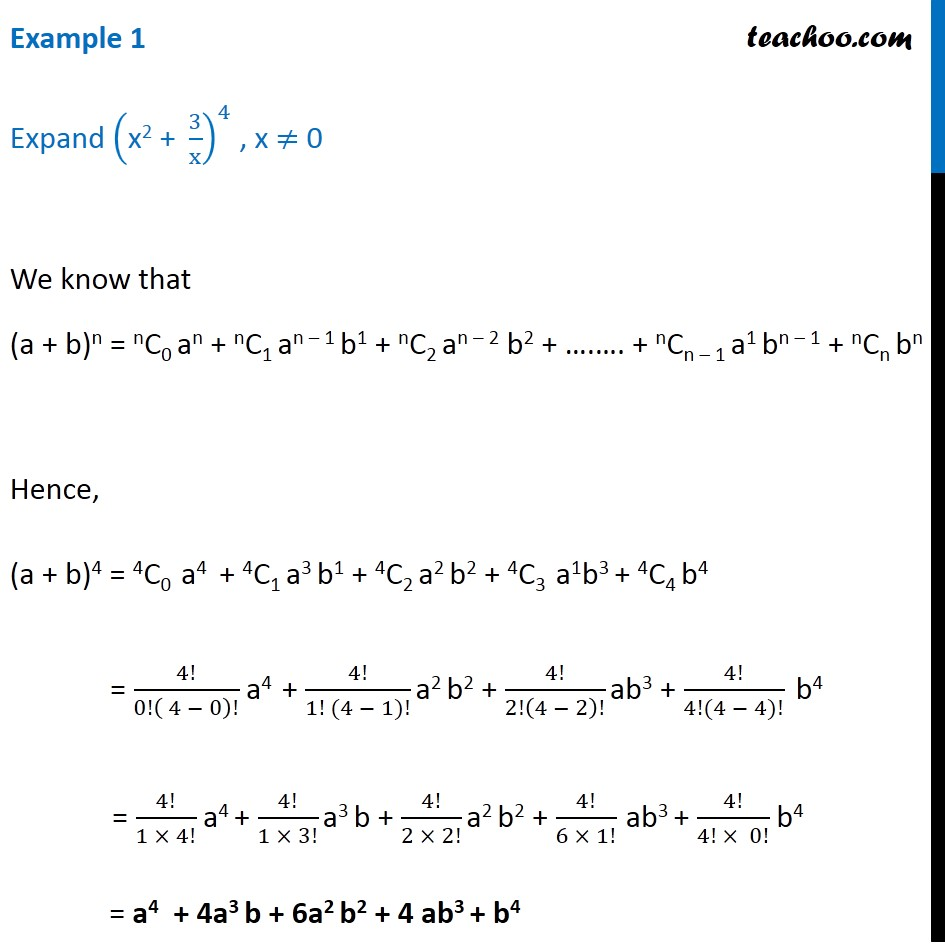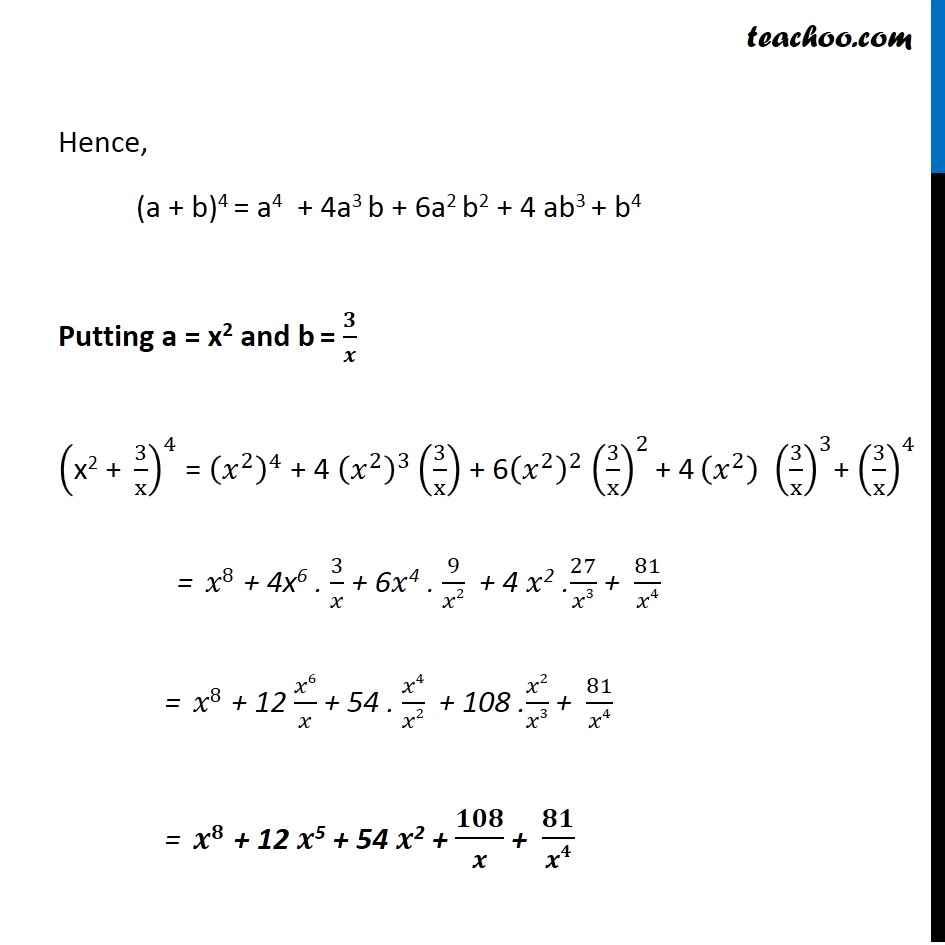Expansion

Chapter 7 Class 11 Binomial Theorem
Concept wiseLearn in your speed, with individual attention - Teachoo Maths 1-on-1 Class

### Transcript

Example 1 Expand ("x2 + " 3/x)^4 , x ≠ 0 We know that (a + b)n = nC0 an + nC1 an – 1 b1 + nC2 an – 2 b2 + ….…. + nCn – 1 a1 bn – 1 + nCn bn Hence, (a + b)4 = 4C0 a4 + 4C1 a3 b1 + 4C2 a2 b2 + 4C3 a1b3 + 4C4 b4 = 4!/0!( 4 − 0)! a4 + 4!/(1! (4 − 1)!) a2 b2 + 4!/2!(4 − 2)! ab3 + 4!/(4!(4 − 4)!) b4 = 4!/(1 × 4!) a4 + 4!/(1 × 3!) a3 b + 4!/(2 × 2!) a2 b2 + 4!/(6 × 1!) ab3 + 4!/(4! × 0!) b4 = a4 + 4a3 b + 6a2 b2 + 4 ab3 + b4 Hence, (a + b)4 = a4 + 4a3 b + 6a2 b2 + 4 ab3 + b4 Putting a = x2 and b = 𝟑/𝒙 ("x2 + " 3/x)^4 = (𝑥^2 )^4 + 4 (𝑥^2 )^3 (3/x) + 6(𝑥^2 )^2 (3/x)^2 + 4 (𝑥^2 ) (3/x)^3+ (3/x)^4 = 𝑥8 + 4x6 . 3/𝑥 + 6𝑥4 . 9/𝑥2 + 4 𝑥2 . 27/𝑥3 + 81/𝑥4 = 𝑥8 + 12 𝑥6/𝑥 + 54 . 𝑥4/𝑥2 + 108 . 𝑥2/𝑥3 + 81/𝑥4 = 𝒙𝟖 + 12 𝒙5 + 54 𝒙2 + 𝟏𝟎𝟖/𝒙 + 𝟖𝟏/𝒙𝟒